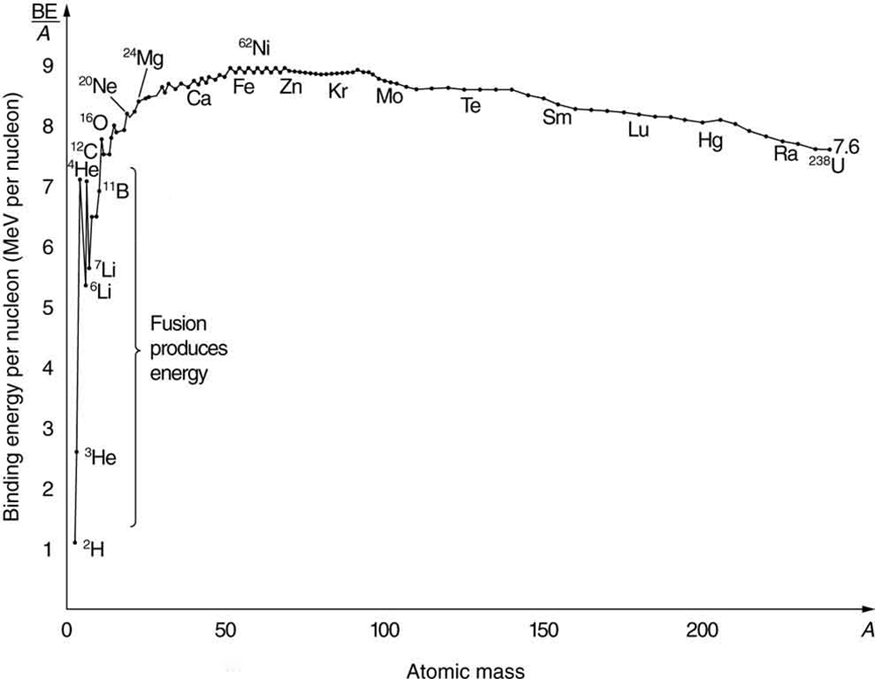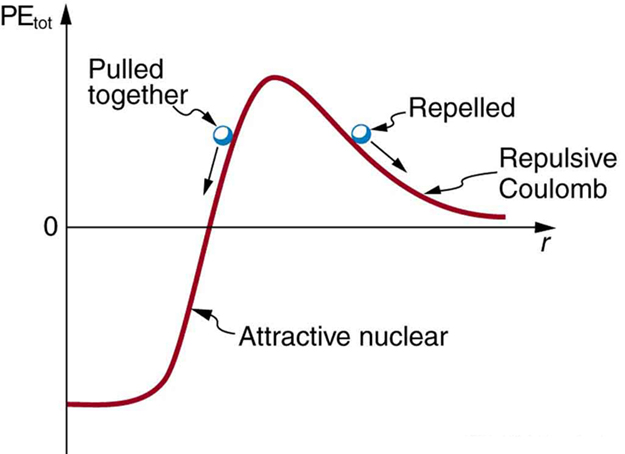# 32.5 Fusion

 Page 1 / 12
• Define nuclear fusion.
• Discuss processes to achieve practical fusion energy generation.

While basking in the warmth of the summer sun, a student reads of the latest breakthrough in achieving sustained thermonuclear power and vaguely recalls hearing about the cold fusion controversy. The three are connected. The Sun’s energy is produced by nuclear fusion (see [link] ). Thermonuclear power is the name given to the use of controlled nuclear fusion as an energy source. While research in the area of thermonuclear power is progressing, high temperatures and containment difficulties remain. The cold fusion controversy centered around unsubstantiated claims of practical fusion power at room temperatures.

Nuclear fusion is a reaction in which two nuclei are combined, or fused , to form a larger nucleus. We know that all nuclei have less mass than the sum of the masses of the protons and neutrons that form them. The missing mass times ${c}^{2}$ equals the binding energy of the nucleus—the greater the binding energy, the greater the missing mass. We also know that $\text{BE}/A$ , the binding energy per nucleon, is greater for medium-mass nuclei and has a maximum at Fe (iron). This means that if two low-mass nuclei can be fused together to form a larger nucleus, energy can be released. The larger nucleus has a greater binding energy and less mass per nucleon than the two that combined. Thus mass is destroyed in the fusion reaction, and energy is released (see [link] ). On average, fusion of low-mass nuclei releases energy, but the details depend on the actual nuclides involved.Fusion of light nuclei to form medium-mass nuclei destroys mass, because BE / A size 12{"BE"/A} {} is greater for the product nuclei. The larger BE / A size 12{"BE"/A} {} is, the less mass per nucleon, and so mass is converted to energy and released in these fusion reactions.

The major obstruction to fusion is the Coulomb repulsion between nuclei. Since the attractive nuclear force that can fuse nuclei together is short ranged, the repulsion of like positive charges must be overcome to get nuclei close enough to induce fusion. [link] shows an approximate graph of the potential energy between two nuclei as a function of the distance between their centers. The graph is analogous to a hill with a well in its center. A ball rolled from the right must have enough kinetic energy to get over the hump before it falls into the deeper well with a net gain in energy. So it is with fusion. If the nuclei are given enough kinetic energy to overcome the electric potential energy due to repulsion, then they can combine, release energy, and fall into a deep well. One way to accomplish this is to heat fusion fuel to high temperatures so that the kinetic energy of thermal motion is sufficient to get the nuclei together.Potential energy between two light nuclei graphed as a function of distance between them. If the nuclei have enough kinetic energy to get over the Coulomb repulsion hump, they combine, release energy, and drop into a deep attractive well. Tunneling through the barrier is important in practice. The greater the kinetic energy and the higher the particles get up the barrier (or the lower the barrier), the more likely the tunneling.

write an expression for a plane progressive wave moving from left to right along x axis and having amplitude 0.02m, frequency of 650Hz and speed if 680ms-¹
how does a model differ from a theory
what is vector quantity
Vector quality have both direction and magnitude, such as Force, displacement, acceleration and etc.
Besmellah
Is the force attractive or repulsive between the hot and neutral lines hung from power poles? Why?
what's electromagnetic induction
electromagnetic induction is a process in which conductor is put in a particular position and magnetic field keeps varying.
Lukman
wow great
Salaudeen
what is mutual induction?
je
mutual induction can be define as the current flowing in one coil that induces a voltage in an adjacent coil.
Johnson
how to undergo polarization
show that a particle moving under the influence of an attractive force mu/y³ towards the axis x. show that if it be projected from the point (0,k) with the component velocities U and V parallel to the axis of x and y, it will not strike the axis of x unless u>v²k² and distance uk²/√u-vk as origin
show that a particle moving under the influence of an attractive force mu/y^3 towards the axis x. show that if it be projected from the point (0,k) with the component velocities U and V parallel to the axis of x and y, it will not strike the axis of x unless u>v^2k^2 and distance uk^2/√u-k as origin
No idea.... Are you even sure this question exist?
Mavis
I can't even understand the question
yes it was an assignment question "^"represent raise to power pls
Gabriel
Gabriel
An engineer builds two simple pendula. Both are suspended from small wires secured to the ceiling of a room. Each pendulum hovers 2 cm above the floor. Pendulum 1 has a bob with a mass of 10kg . Pendulum 2 has a bob with a mass of 100 kg . Describe how the motion of the pendula will differ if the bobs are both displaced by 12º .
no ideas
Augstine
if u at an angle of 12 degrees their period will be same so as their velocity, that means they both move simultaneously since both both hovers at same length meaning they have the same length
Modern cars are made of materials that make them collapsible upon collision. Explain using physics concept (Force and impulse), how these car designs help with the safety of passengers.
calculate the force due to surface tension required to support a column liquid in a capillary tube 5mm. If the capillary tube is dipped into a beaker of water
find the time required for a train Half a Kilometre long to cross a bridge almost kilometre long racing at 100km/h
method of polarization
Ajayi
What is atomic number?
The number of protons in the nucleus of an atom
Deborah
type of thermodynamics
oxygen gas contained in a ccylinder of volume has a temp of 300k and pressure 2.5×10Nm
why the satellite does not drop to the earth explain
what is a matter
Yinka
what is matter
Yinka
what is matter
Yinka
what is a matter
Yinka
I want the nuclear physics conversation
Mohamed
because space is a vacuum and anything outside the earth 🌎 can not come back without an act of force applied to it to leave the vacuum and fall down to the earth with a maximum force length of 30kcm per second
Clara

#### Get Jobilize Job Search Mobile App in your pocket Now!ByBy Brooke DelaneyBy RhodesBy OpenStaxBy OpenStaxBy OpenStaxBy OpenStaxBy Yacoub JayoghliBy OpenStaxBy OpenStaxBy Jonathan Long